# RS Aggarwal Solutions for Class 7 Maths Chapter 14 Properties of Parallel Lines

RS Aggarwal Solutions for Class 7 Maths Chapter 14 – Properties of Parallel Lines is the best study material for those students who are finding difficulties in solving problems. Our specialist teachers formulate these exercises to help you with your exam preparation to achieve good marks in Maths. These solutions can help students clear doubts quickly and help in learning the topic effectively. Students who wish to score good marks in Maths practise RS Aggarwal Class 7 Solutions.

These Properties of Parallel Lines solutions are available for download in pdf format and provide solutions to all the questions provided in Class 7 Maths Chapter 14 wherein problems are solved step by step with detailed explanations. Download pdf of Class 7 Chapter 14 in their respective links.

## Download PDF of RS Aggarwal Solutions for Class 7 Maths Chapter 14 – Properties of Parallel lines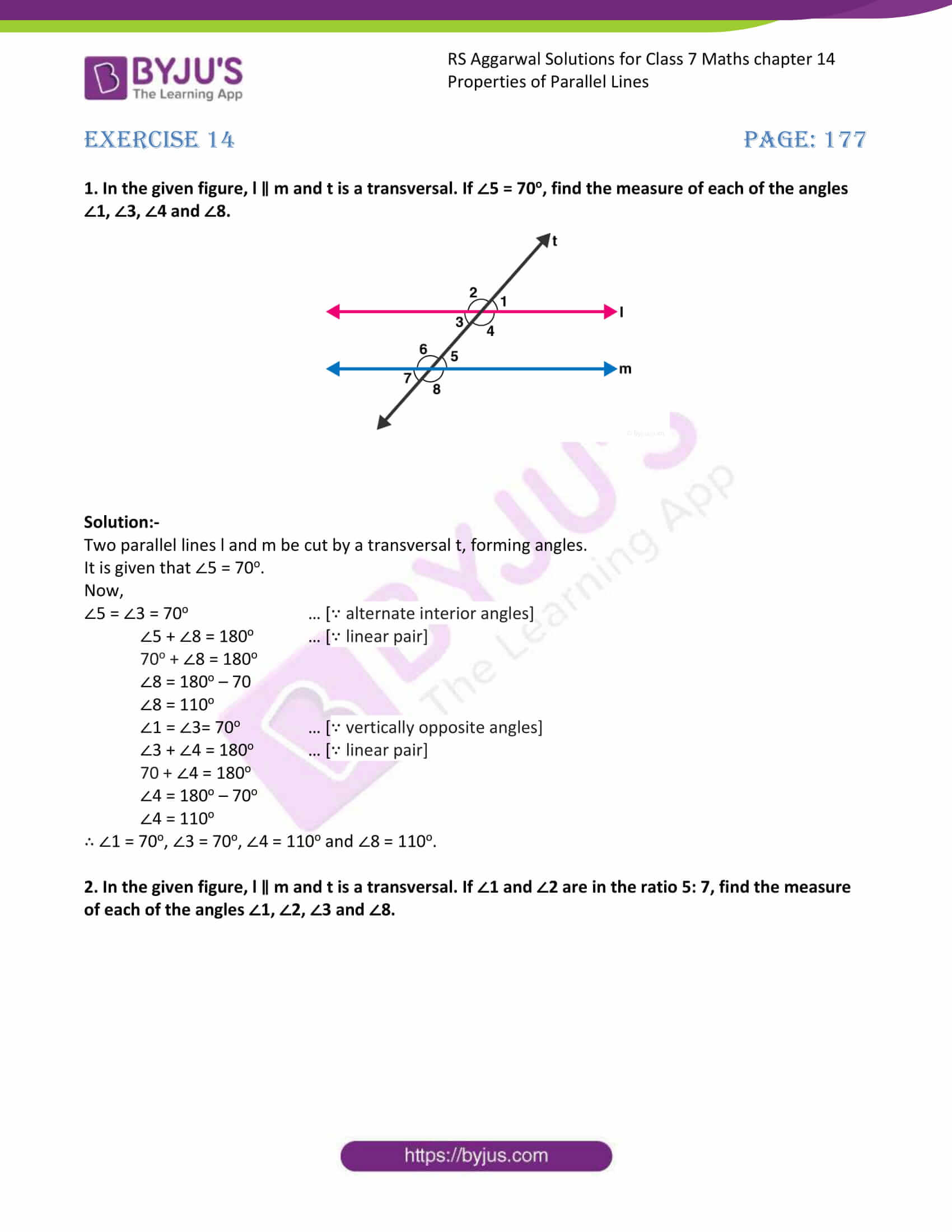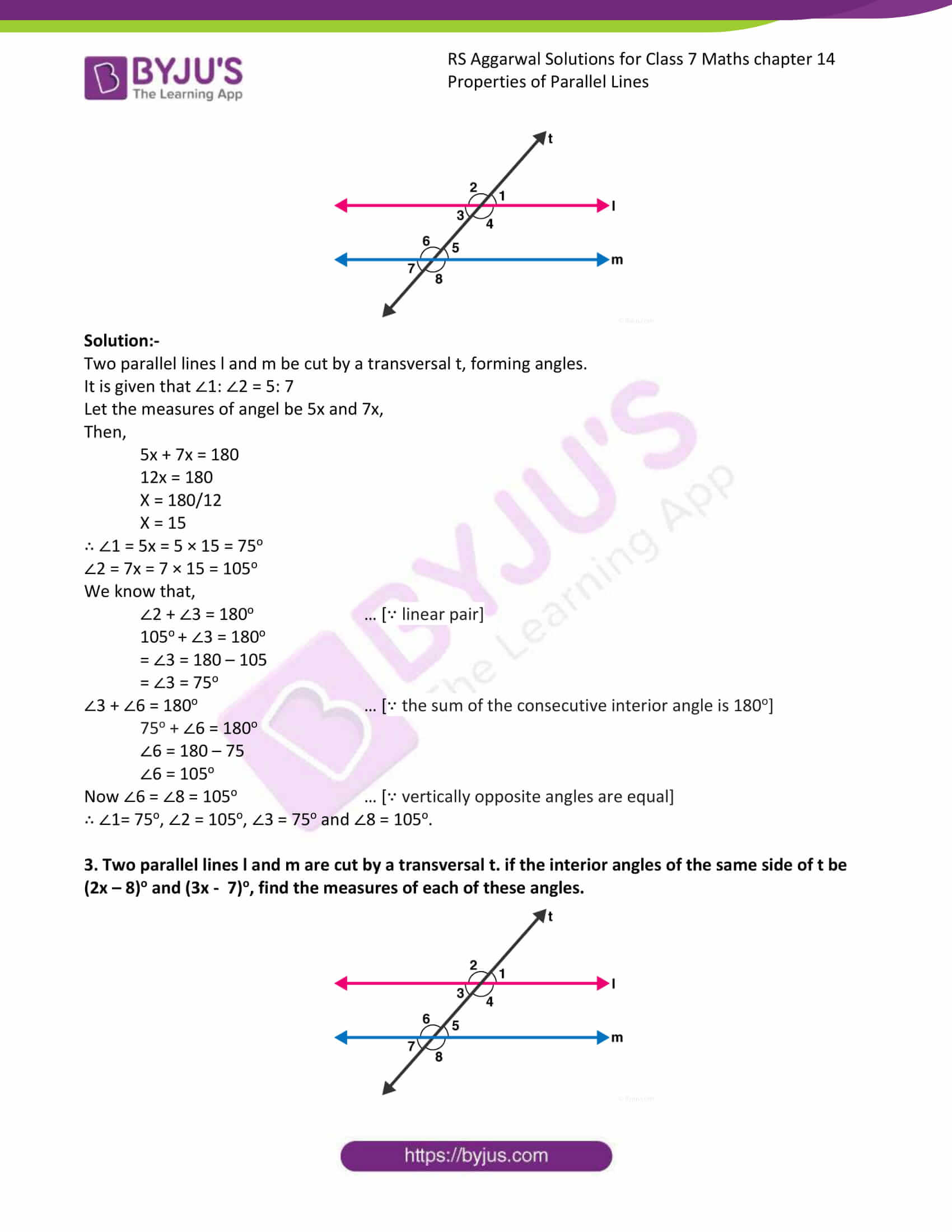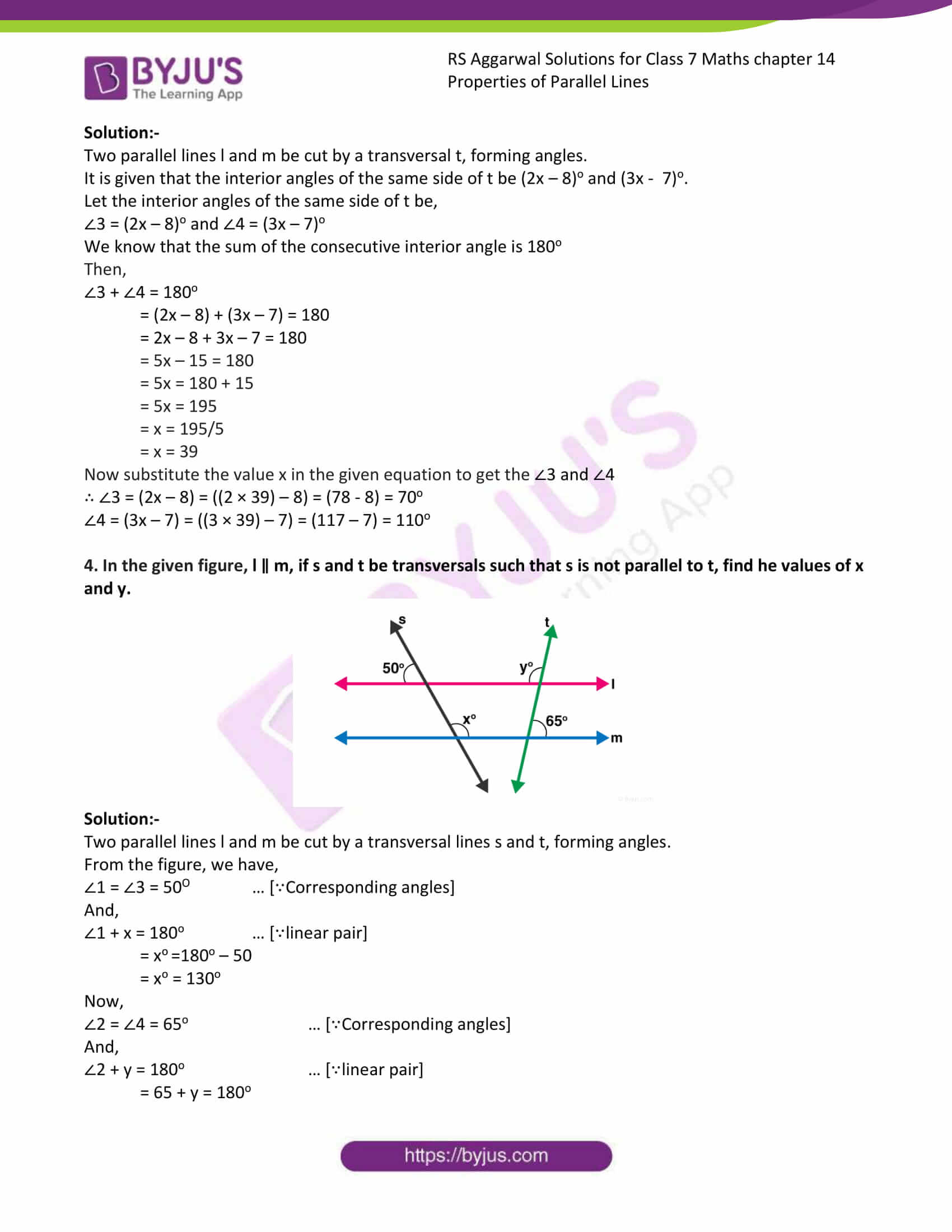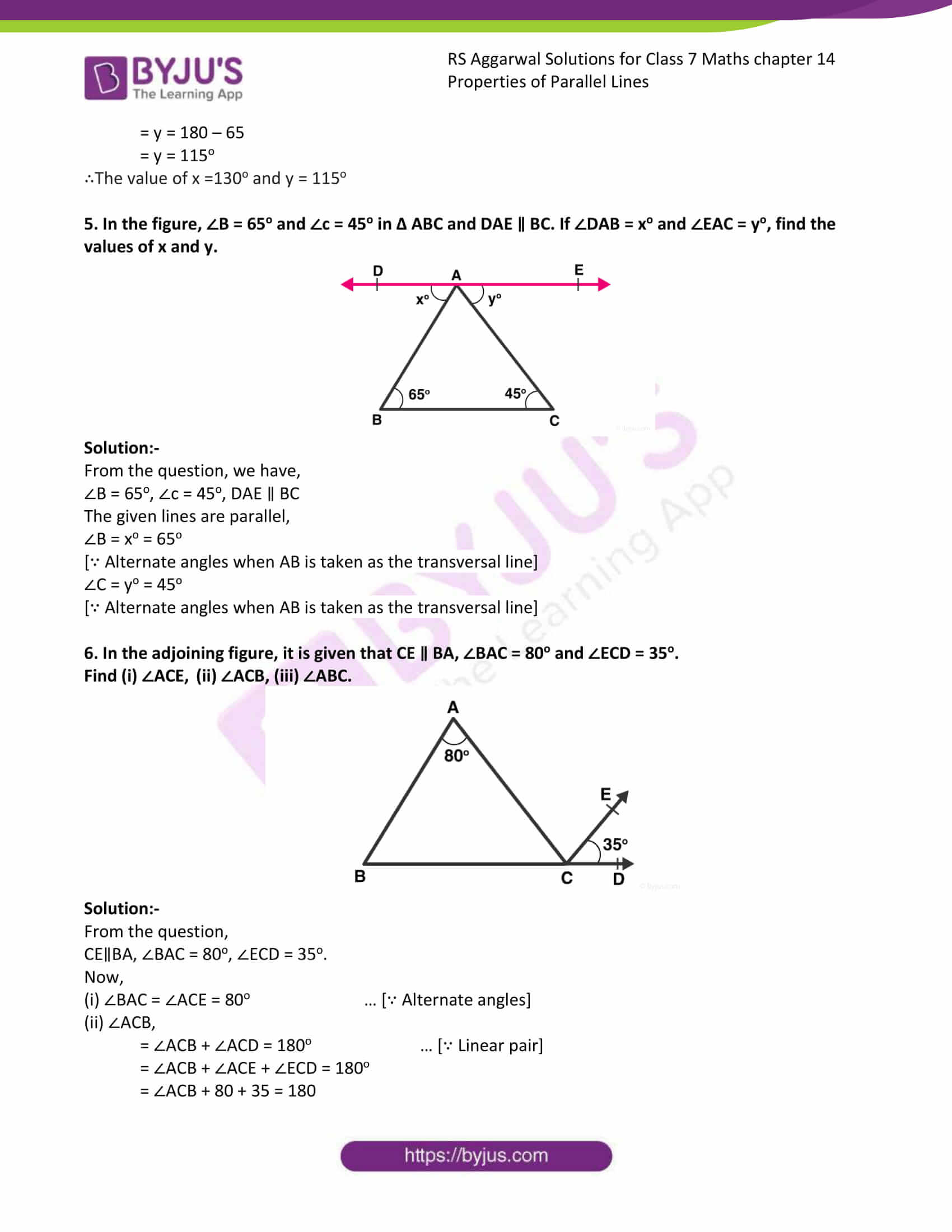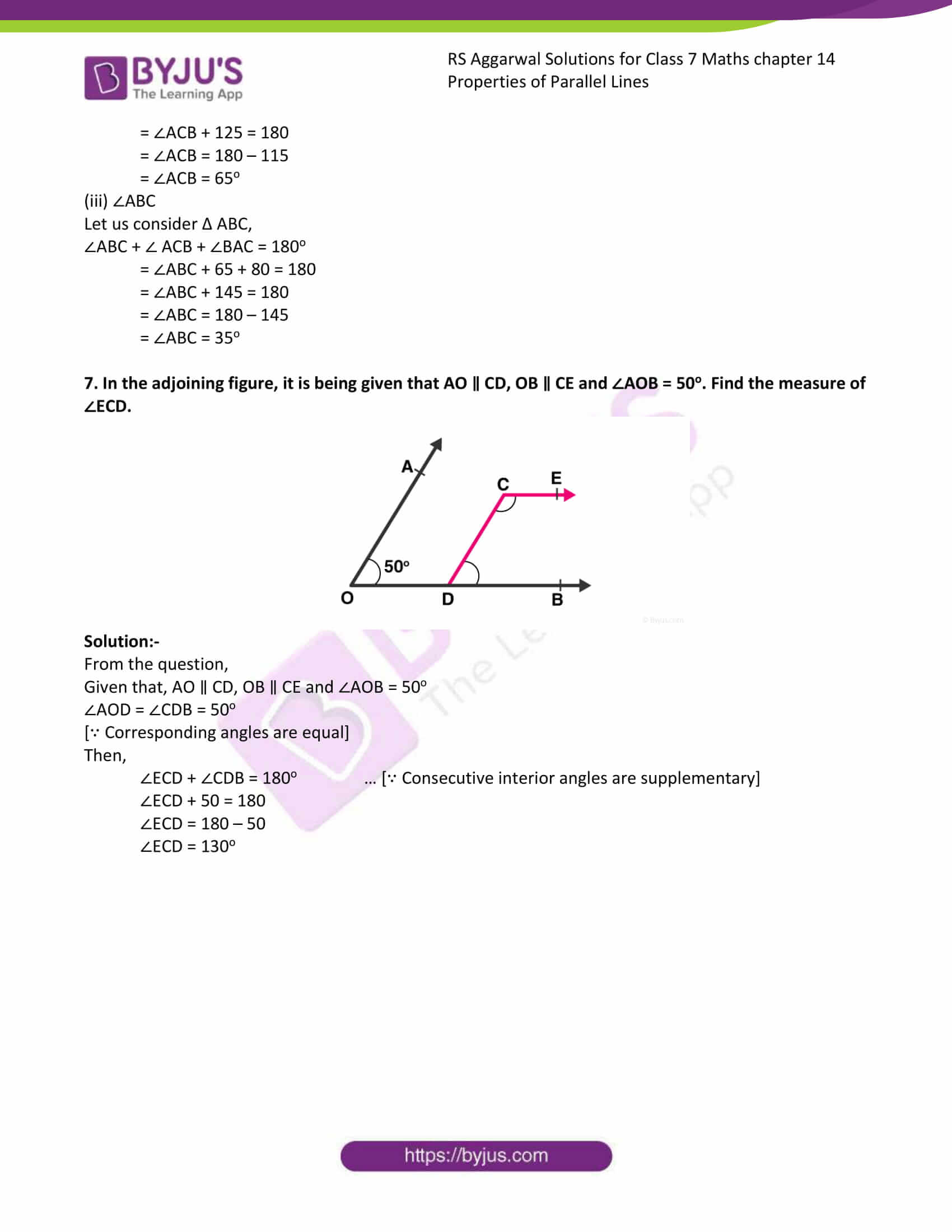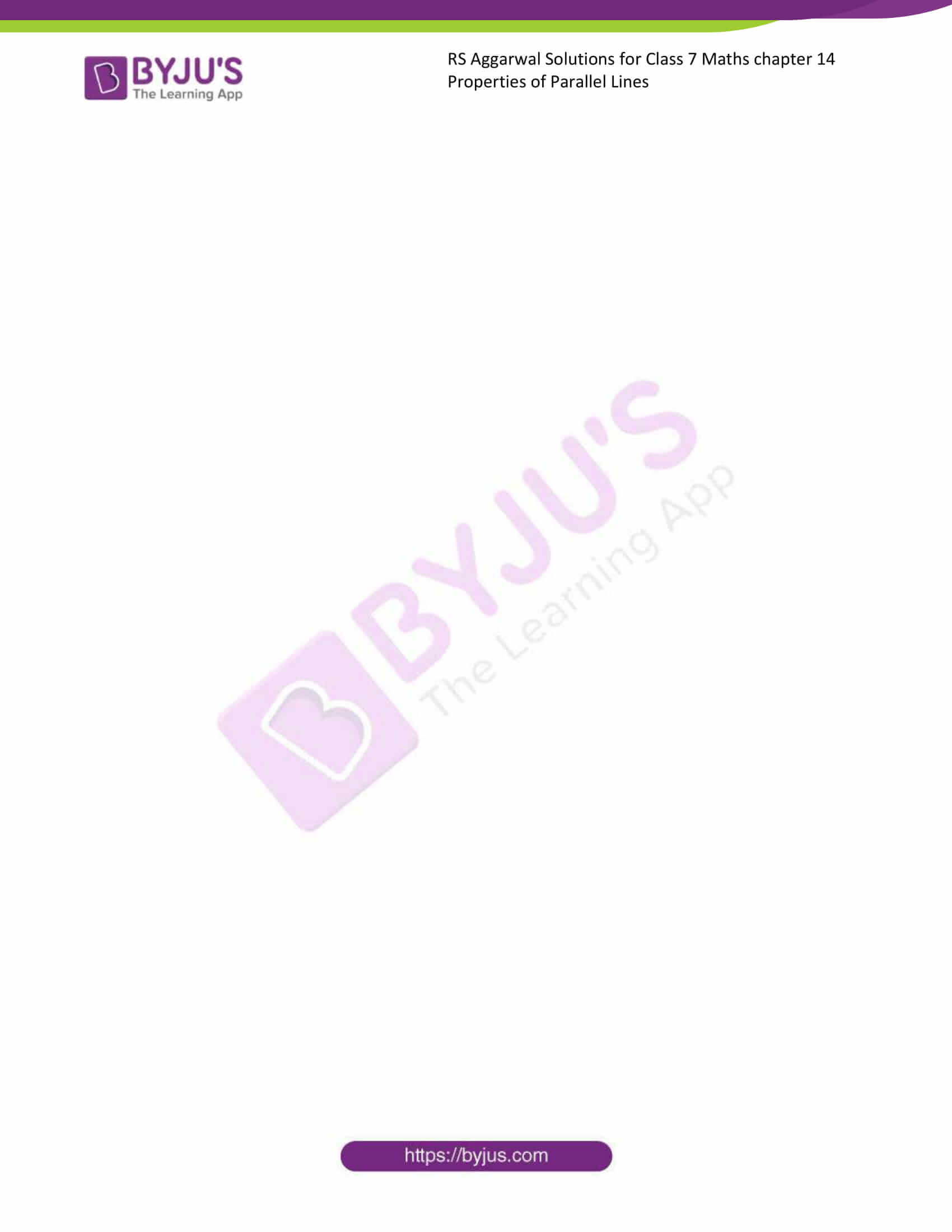### Access answer to chapter 14 – Properties of Parallel Lines

Exercise 14

1. In the given figure, l ∥ m and t is a transversal. If ∠5 = 70o, find the measure of each of the angles ∠1, ∠3, ∠4 and ∠8.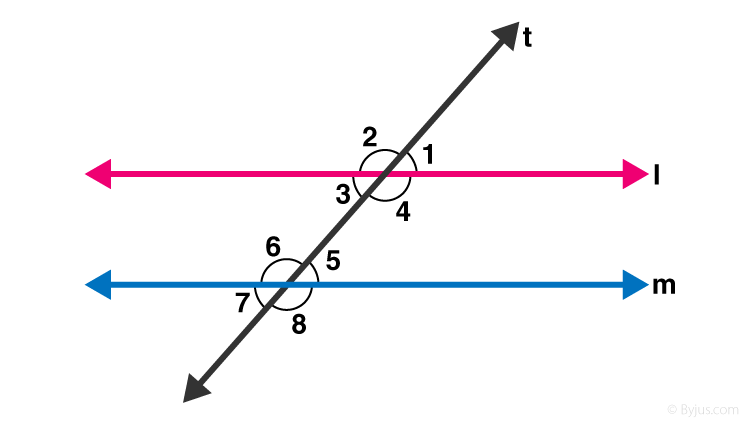Solution:-

Two parallel lines l and m be cut by a transversal t, forming angles.

It is given that ∠5 = 70o.

Now,

∠5 = ∠3 = 70o … [∵ alternate interior angles]

∠5 + ∠8 = 180o … [∵ linear pair]

70o + ∠8 = 180o

∠8 = 180o – 70

∠8 = 110o

∠1 = ∠3= 70o … [∵ vertically opposite angles]

∠3 + ∠4 = 180o … [∵ linear pair]

70 + ∠4 = 180o

∠4 = 180o – 70o

∠4 = 110o

∴ ∠1 = 70o, ∠3 = 70o, ∠4 = 110o and ∠8 = 110o.

2. In the given figure, l ∥ m and t is a transversal. If ∠1 and ∠2 are in the ratio 5: 7, find the measure of each of the angles ∠1, ∠2, ∠3 and ∠8.Solution:-

Two parallel lines l and m be cut by a transversal t, forming angles.

It is given that ∠1: ∠2 = 5: 7

Let the measures of angel be 5x and 7x,

Then,

5x + 7x = 180

12x = 180

X = 180/12

X = 15

∴ ∠1 = 5x = 5 × 15 = 75o

∠2 = 7x = 7 × 15 = 105o

We know that,

∠2 + ∠3 = 180o … [∵ linear pair]

105o + ∠3 = 180o

= ∠3 = 180 – 105

= ∠3 = 75o

∠3 + ∠6 = 180o … [∵ the sum of the consecutive interior angle is 180o]

75o + ∠6 = 180o

∠6 = 180 – 75

∠6 = 105o

Now ∠6 = ∠8 = 105o … [∵ vertically opposite angles are equal]

∴ ∠1= 75o, ∠2 = 105o, ∠3 = 75o and ∠8 = 105o.

3. Two parallel lines l and m are cut by a transversal t. if the interior angles of the same side of t be (2x – 8)o and (3x – 7)o, find the measures of each of these angles.Solution:-

Two parallel lines l and m be cut by a transversal t, forming angles.

It is given that the interior angles of the same side of t be (2x – 8)o and (3x – 7)o.

Let the interior angles of the same side of t be,

∠3 = (2x – 8)o and ∠4 = (3x – 7)o

We know that the sum of the consecutive interior angle is 180o

Then,

∠3 + ∠4 = 180o

= (2x – 8) + (3x – 7) = 180

= 2x – 8 + 3x – 7 = 180

= 5x – 15 = 180

= 5x = 180 + 15

= 5x = 195

= x = 195/5

= x = 39

Now substitute the value x in the given equation to get the ∠3 and ∠4

∴ ∠3 = (2x – 8) = ((2 × 39) – 8) = (78 – 8) = 70o

∠4 = (3x – 7) = ((3 × 39) – 7) = (117 – 7) = 110o

4. In the given figure, l ∥ m, if s and t be transversals such that s is not parallel to t, find he values of x and y.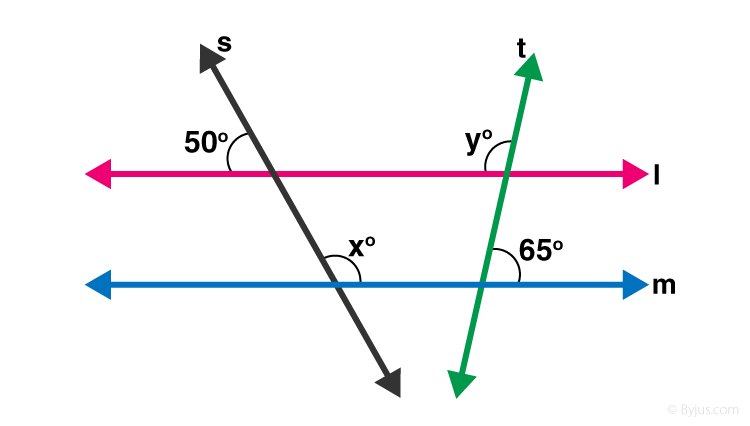Solution:-

Two parallel lines l and m be cut by a transversal lines s and t, forming angles.

From the figure, we have,

∠1 = ∠3 = 50O … [∵Corresponding angles]

And,

∠1 + x = 180o … [∵linear pair]

= xo =180o – 50

= xo = 130o

Now,

∠2 = ∠4 = 65o … [∵Corresponding angles]

And,

∠2 + y = 180o … [∵linear pair]

= 65 + y = 180o

= y = 180 – 65

= y = 115o

∴The value of x =130o and y = 115o

5. In the figure, ∠B = 65o and ∠c = 45o in Δ ABC and DAE ∥ BC. If ∠DAB = xo and ∠EAC = yo, find the values of x and y.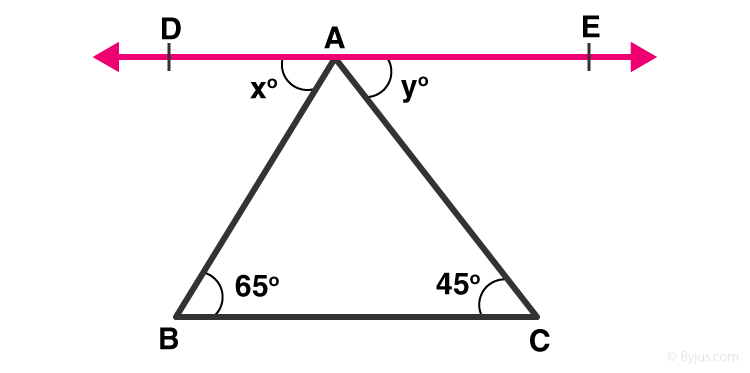Solution:-

From the question, we have,

∠B = 65o, ∠c = 45o, DAE ∥ BC

The given lines are parallel,

∠B = xo = 65o

[∵ Alternate angles when AB is taken as the transversal line]

∠C = yo = 45o

[∵ Alternate angles when AB is taken as the transversal line]

6. In the adjoining figure, it is given that CE ∥ BA, ∠BAC = 80o and ∠ECD = 35o.

Find (i) ∠ACE, (ii) ∠ACB, (iii) ∠ABC.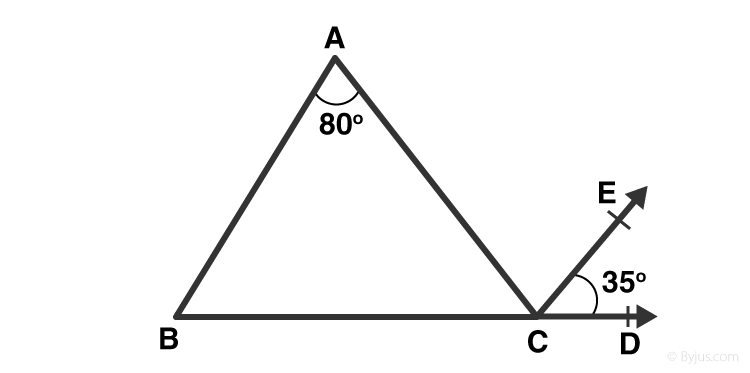Solution:-

From the question,

CE∥BA, ∠BAC = 80o, ∠ECD = 35o.

Now,

(i) ∠BAC = ∠ACE = 80o … [∵ Alternate angles]

(ii) ∠ACB,

= ∠ACB + ∠ACD = 180o … [∵ Linear pair]

= ∠ACB + ∠ACE + ∠ECD = 180o

= ∠ACB + 80 + 35 = 180

= ∠ACB + 125 = 180

= ∠ACB = 180 – 115

= ∠ACB = 65o

(iii) ∠ABC

Let us consider Δ ABC,

∠ABC + ∠ ACB + ∠BAC = 180o

= ∠ABC + 65 + 80 = 180

= ∠ABC + 145 = 180

= ∠ABC = 180 – 145

= ∠ABC = 35o

7. In the adjoining figure, it is being given that AO ∥ CD, OB ∥ CE and ∠AOB = 50o. Find the measure of ∠ECD.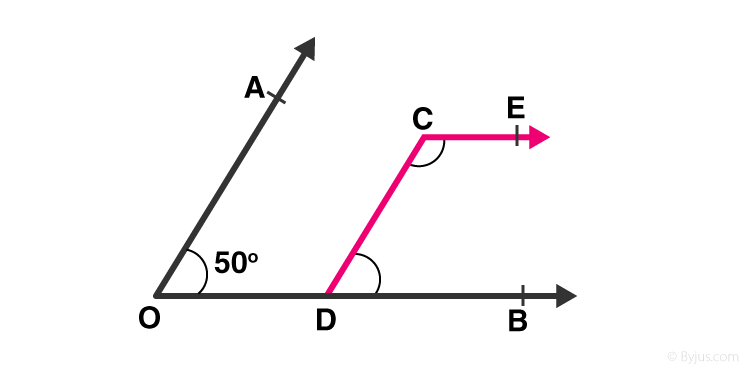Solution:-

From the question,

Given that, AO ∥ CD, OB ∥ CE and ∠AOB = 50o

∠AOD = ∠CDB = 50o

[∵ Corresponding angles are equal]

Then,

∠ECD + ∠CDB = 180o … [∵ Consecutive interior angles are supplementary]

∠ECD + 50 = 180

∠ECD = 180 – 50

∠ECD = 130o

## RS Aggarwal Solutions for Class 7 Maths Chapter 14 – Properties of Parallel Lines

Chapter 14 – Properties of Parallel Lines contains 1 exercise and the RS Aggarwal Solutions available on this page provide solutions to the questions present in the exercises. Now, let us have a look at some of the concepts discussed in this chapter.

• Parallel Lines
• Distance Between Parallel Lines
• Transversal
• Angles Formed When a Transversal CutsTwo Parallel Lines

### Chapter Brief of RS Aggarwal Solutions for Class 7 Maths Chapter 14 – Properties of Parallel Lines

RS Aggarwal Solutions for Class 7 Maths Chapter 14 – Properties of Parallel Lines. The chapter covers types of angles forming when a transversal cuts two parallel lines and also properties of angles.The solutions are solved in such a way that students will understand clearly.